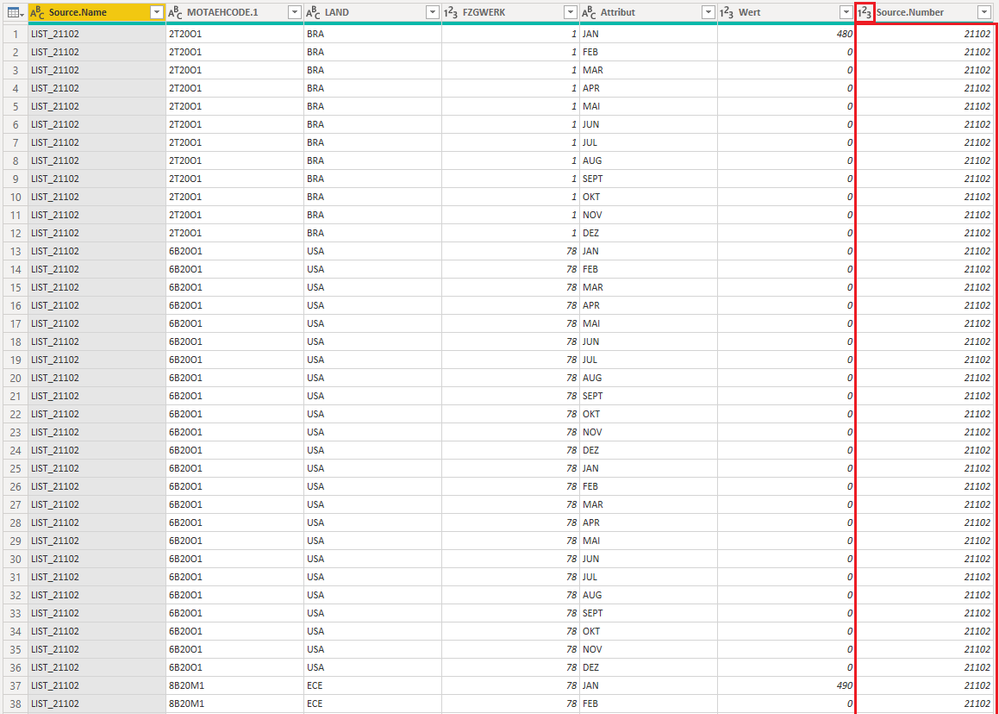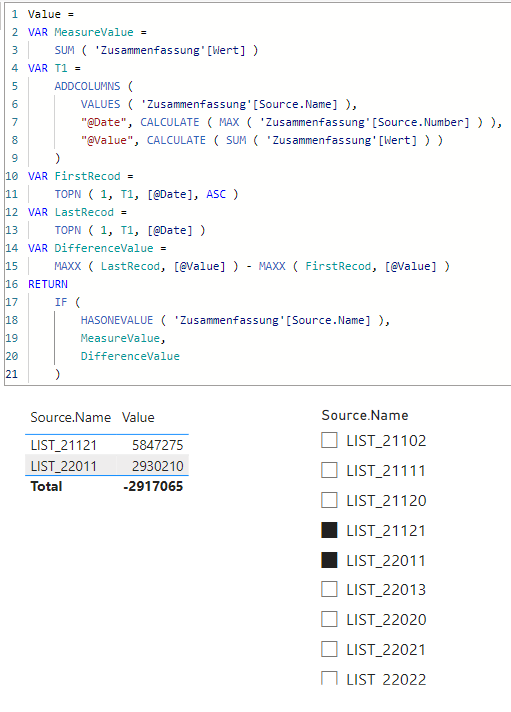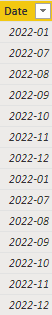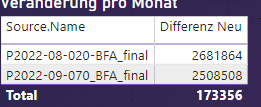cancel
Showing results for
Did you mean:Helper I

## How to get the difference between more rows?

Hey guys,

i need your help please i hava a huge table with lot of data (many excel files in one folder) and i want the differences between the Sources (excelfiles).

I have found something which is almost good for that what i want in the Quick Measures:

Value difference from LIST_211022_MOT_PROG_P2022-01_final.xlsx =
VAR __BASELINE_VALUE =
CALCULATE(
SUM('Zusammenfassung'[Value]),
'Zusammenfassung'[Source.Name]
IN { "LIST_211022_MOT_PROG_P2022-01_final.xlsx" }
)
VAR __MEASURE_VALUE = SUM('Zusammenfassung'[Value])
RETURN
IF(NOT ISBLANK(__MEASURE_VALUE), __MEASURE_VALUE - __BASELINE_VALUE)

But the problem here ist that the Source is not dinamic, i have to filter between the differen excel files and need that den differences.

Almost like the function in the pivot tabel (show values as difference).

2 ACCEPTED SOLUTIONSSuper User

@KimBoehmer
If you want to follow my suggestion and disply the results in a tablevisual, then place the Sorce Name column in the table along with the following measure. From the visual format options, activate the total and rename it "Difference"

``````Value =
VAR MeasureValue =
SUM ( 'Zusammenfassung'[Value] )
VAR T1 =
VALUES ( 'Zusammenfassung'[Source.Name] ),
"@Value", CALCULATE ( SUM ( 'Zusammenfassung'[Value] ) )
)
VAR DifferenceValue =
MAXX ( T1, [@Value] ) - MINX ( T1, [@Value] )
RETURN
IF (
HASONEVALUE ( 'Zusammenfassung'[Source.Name] ),
MeasureValue,
DifferenceValue
)``````

If you wish to display the difference in a seperate chart, then place the following measure in the chart ALONE (don't place any column along with it in the same chart)

``````Value difference =
VAR T1 =
VALUES ( 'Zusammenfassung'[Source.Name] ),
"@Value", CALCULATE ( SUM ( 'Zusammenfassung'[Value] ) )
)
RETURN
MAXX ( T1, [@Value] ) - MINX ( T1, [@Value] )``````Super User

Hi @KimBoehmer
Please refer to attached sample file with the solution. I've created a new column using power query to get the Source Number for sorting purposes.``````Value =
VAR MeasureValue =
SUM ( 'Zusammenfassung'[Wert] )
VAR T1 =
VALUES ( 'Zusammenfassung'[Source.Name] ),
"@Date", CALCULATE ( MAX ( 'Zusammenfassung'[Source.Number] ) ),
"@Value", CALCULATE ( SUM ( 'Zusammenfassung'[Wert] ) )
)
VAR FirstRecod =
TOPN ( 1, T1, [@Date], ASC )
VAR LastRecod =
TOPN ( 1, T1, [@Date] )
VAR DifferenceValue =
MAXX ( LastRecod, [@Value] ) - MAXX ( FirstRecod, [@Value] )
RETURN
IF (
HASONEVALUE ( 'Zusammenfassung'[Source.Name] ),
MeasureValue,
DifferenceValue
)``````
22 REPLIES 22Helper I

Hi, i did it but get no result:The error is gone but there is no outputHelper I

Here the example ( the older version is on top, the newest version on the bottom):Normaly the difference between these two should be negative and not positiv.

Do you know what i mean?